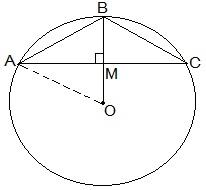Guru

# Three girls Reshma, Salma and Mandip are playing a game by standing on a circle of radius 5m drawn in a park. Reshma throws a ball to Salma, Salma to Mandip, Mandip to Reshma. If the distance between Reshma and Salma and between Salma and Mandip is 6m each, what is the distance between Reshma and Mandip? Q.5

• 0

Yesterday i was doing the question from class 9th ncert book of math of Circles chapter of exercise 10.4  What is the easiest way for solving it because i was not able to do this question please help me for solving this question no 5 Three girls Reshma, Salma and Mandip are playing a game by standing on a circle of radius 5m drawn in a park. Reshma throws a ball to Salma, Salma to Mandip, Mandip to Reshma. If the distance between Reshma and Salma and between Salma and Mandip is 6m each, what is the distance between Reshma and Mandip?

Share

1.Let the positions of Reshma, Salma and Mandip be represented as A, B and C respectively.

From the question, we know that AB = BC = 6cm.

So, the radius of the circle i.e. OA = 5cm

Now, draw a perpendicular BM ⊥ AC.

Since AB = BC, ABC can be considered as an isosceles triangle. M is mid-point of AC. BM is the perpendicular bisector of AC and thus it passes through the centre of the circle.

Now,

let AM = y and

OM = x

So, BM will be = (5-x).

By applying Pythagorean theorem in ΔOAM we get,

OA2 = OM2 +AM2

⇒ 52 = x2 +y2 — (i)

Again, by applying Pythagorean theorem in ΔAMB,

AB2 = BM2 +AM2

⇒ 62 = (5-x)2+y2 — (ii)

Subtracting equation (i) from equation (ii), we get

36-25 = (5-x)2 +y2 -x2-y2

Now, solving this equation we get the value of x as

x = 7/5

Substituting the value of x in equation (i), we get

y2 +(49/25) = 25

⇒ y2 = 25 – (49/25)

Solving it we get the value of y as

y = 24/5

Thus,

AC = 2×AM

= 2×y

= 2×(24/5) m

AC = 9.6 m

So, the distance between Reshma and Mandip is 9.6 m.

• 0